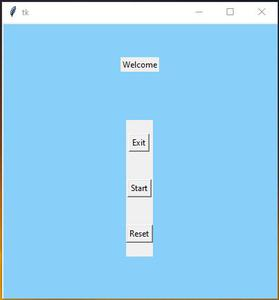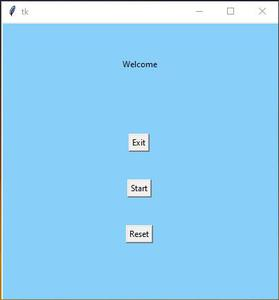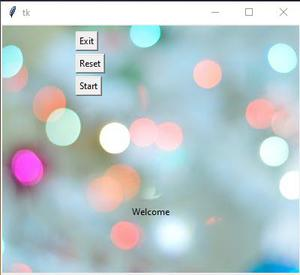# How To Use Images as Backgrounds in Tkinter?

Prerequisite: Python GUI – tkinter , Frame

In this article, We are going to write a program use image in the background. In Tkinter, there is no in-built function for images, so that it can be used as a background image. It can be done with various methods:

Method 1: Using photoimage methods.

When it comes to GUI based application, images play a vital role. From the application icon to animation, it’s useful.

To display images in labels, buttons, canvases, and text widgets, the PhotoImage class is used, which is present in Tkinter package.

Code:

## Python3

 `# Import module  ` `from` `tkinter ``import` `*` ` `  `# Create object  ` `root ``=` `Tk() ` ` `  `# Adjust size  ` `root.geometry(``"400x400"``) ` ` `  `# Add image file ` `bg ``=` `PhotoImage(``file` `=` `"Your_image.png"``) ` ` `  `# Show image using label ` `label1 ``=` `Label( root, image ``=` `bg) ` `label1.place(x ``=` `0``, y ``=` `0``) ` ` `  `label2 ``=` `Label( root, text ``=` `"Welcome"``) ` `label2.pack(pady ``=` `50``) ` ` `  `# Create Frame ` `frame1 ``=` `Frame(root) ` `frame1.pack(pady ``=` `20` `) ` ` `  `# Add buttons ` `button1 ``=` `Button(frame1,text``=``"Exit"``) ` `button1.pack(pady``=``20``) ` ` `  `button2 ``=` `Button( frame1, text ``=` `"Start"``) ` `button2.pack(pady ``=` `20``) ` ` `  `button3 ``=` `Button( frame1, text ``=` `"Reset"``) ` `button3.pack(pady ``=` `20``) ` ` `  `# Execute tkinter ` `root.mainloop()`

Output:As you can see the background color of buttons and labels have different from the image color.

The solution is to set the background color of buttons and label it as the color of the image with this color  “#88cffa” .

## Python3

 `# Import module  ` `from` `tkinter ``import` `*` ` `  `# Create object  ` `root ``=` `Tk() ` ` `  `# Adjust size  ` `root.geometry(``"400x400"``) ` ` `  `# Add image file ` `bg ``=` `PhotoImage( ``file` `=` `"Your_img.png"``) ` ` `  `# Show image using label ` `label1 ``=` `Label( root, image ``=` `bg) ` `label1.place(x ``=` `0``,y ``=` `0``) ` ` `  `# Add text ` `label2 ``=` `Label( root, text ``=` `"Welcome"``, ` `               ``bg ``=` `"#88cffa"``) ` ` `  `label2.pack(pady ``=` `50``) ` ` `  `# Create Frame ` `frame1 ``=` `Frame( root, bg ``=` `"#88cffa"``) ` `frame1.pack(pady ``=` `20``) ` ` `  `# Add buttons ` `button1 ``=` `Button( frame1, text ``=` `"Exit"``) ` `button1.pack(pady ``=` `20``) ` ` `  `button2 ``=` `Button( frame1, text ``=` `"Start"``) ` `button2.pack(pady ``=` `20``) ` ` `  `button3 ``=` `Button( frame1, text ``=` `"Reset"``) ` `button3.pack(pady ``=` `20``) ` ` `  `# Execute tkinter ` `root.mainloop()`

Output:Note: This method will not work for multiple colors in image.

Method 2: Using Canvas methods.

Approach:

• Same as above implementation.
• Create Canvas and set width and height.
• Display image using create_image.
• Set text using create_text.
• Create buttons.
• Final step add button using create_window.

Code:

## Python3

 `# Import module  ` `from` `tkinter ``import` `*` ` `  `# Create object  ` `root ``=` `Tk() ` ` `  `# Adjust size  ` `root.geometry(``"400x400"``) ` ` `  `# Add image file ` `bg ``=` `PhotoImage(``file` `=` `"Your_img.png"``) ` ` `  `# Create Canvas ` `canvas1 ``=` `Canvas( root, width ``=` `400``, ` `                 ``height ``=` `400``) ` ` `  `canvas1.pack(fill ``=` `"both"``, expand ``=` `True``) ` ` `  `# Display image ` `canvas1.create_image( ``0``, ``0``, image ``=` `bg,  ` `                     ``anchor ``=` `"nw"``) ` ` `  `# Add Text ` `canvas1.create_text( ``200``, ``250``, text ``=` `"Welcome"``) ` ` `  `# Create Buttons ` `button1 ``=` `Button( root, text ``=` `"Exit"``) ` `button3 ``=` `Button( root, text ``=` `"Start"``) ` `button2 ``=` `Button( root, text ``=` `"Reset"``) ` ` `  `# Display Buttons ` `button1_canvas ``=` `canvas1.create_window( ``100``, ``10``,  ` `                                       ``anchor ``=` `"nw"``, ` `                                       ``window ``=` `button1) ` ` `  `button2_canvas ``=` `canvas1.create_window( ``100``, ``40``, ` `                                       ``anchor ``=` `"nw"``, ` `                                       ``window ``=` `button2) ` ` `  `button3_canvas ``=` `canvas1.create_window( ``100``, ``70``, anchor ``=` `"nw"``, ` `                                       ``window ``=` `button3) ` ` `  `# Execute tkinter ` `root.mainloop() `

Output:Whether you're preparing for your first job interview or aiming to upskill in this ever-evolving tech landscape, GeeksforGeeks Courses are your key to success. We provide top-quality content at affordable prices, all geared towards accelerating your growth in a time-bound manner. Join the millions we've already empowered, and we're here to do the same for you. Don't miss out - check it out now!

Previous
Next